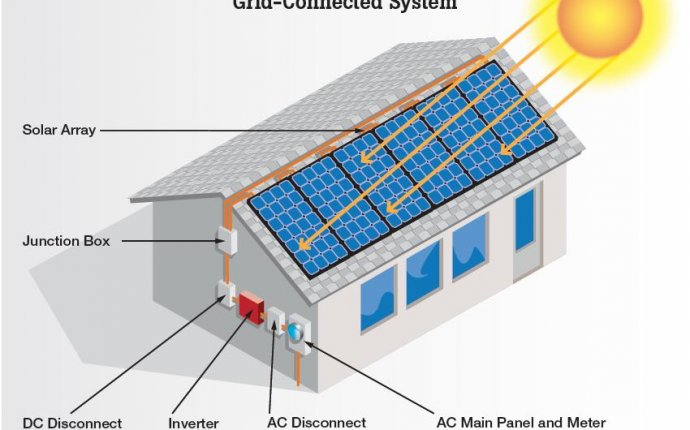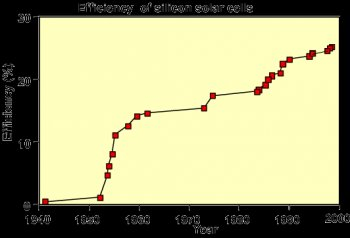## Design of solar Panels

Solar Panels / June 2, 2017

Solar cell design involves specifying the parameters of a solar cell structure in order to maximize efficiency, given a certain set of constraints. These constraints will be defined by the working environment in which solar cells are produced. For example in a commercial environment where the objective is to produce a competitively priced solar cell, the cost of fabricating a particular solar cell structure must be taken into consideration. However, in a research environment where the objective is to produce a highly efficient laboratory-type cell, maximizing efficiency rather than cost, is the main consideration.Evolution of silicon solar cell efficiency.

The theoretical efficiency for photovoltaic conversion is in excess of 86.8% . However, the 86.8% figure uses detailed balance calculations and does not describe device implementation. For silicon solar cells, a more realistic efficiency under one sun operation is about 29% . The maximum efficiency measured for a silicon solar cell is currently 24.7% under AM1.5G. The difference between the high theoretical efficiencies and the efficiencies measured from terrestrial solar cells is due mainly to two factors. The first is that the theoretical maximum efficiency predictions assume that energy from each photon is optimally used, that there are no unabsorbed photons and that each photon is absorbed in a material which has a band gap equal to the photon energy. This is achieved in theory by modeling an infinite stack of solar cells of different band gap materials, each absorbing only the photons which correspond exactly to its band gap.

The second factor is that the high theoretical efficiency predictions assume a high concentration ratio. Assuming that temperature and resistive effects do not dominate in a concentrator solar cell, increasing the light intensity proportionally increases the short-circuit current. Since the open-circuit voltage (Voc) also depends on the short-circuit current, Voc increases logarithmically with light level. Furthermore, since the maximum fill factor (FF) increases with Voc, the maximum possible FF also increases with concentration. The extra Voc and FF increases with concentration which allows concentrators to achieve higher efficiencies.

In designing such single junction solar cells, the principles for maximizing cell efficiency are:

• increasing the amount of light collected by the cell that is turned into carriers;
• increasing the collection of light-generated carriers by the p-n junction;
• minimising the forward bias dark current;

Source: www.pveducation.org